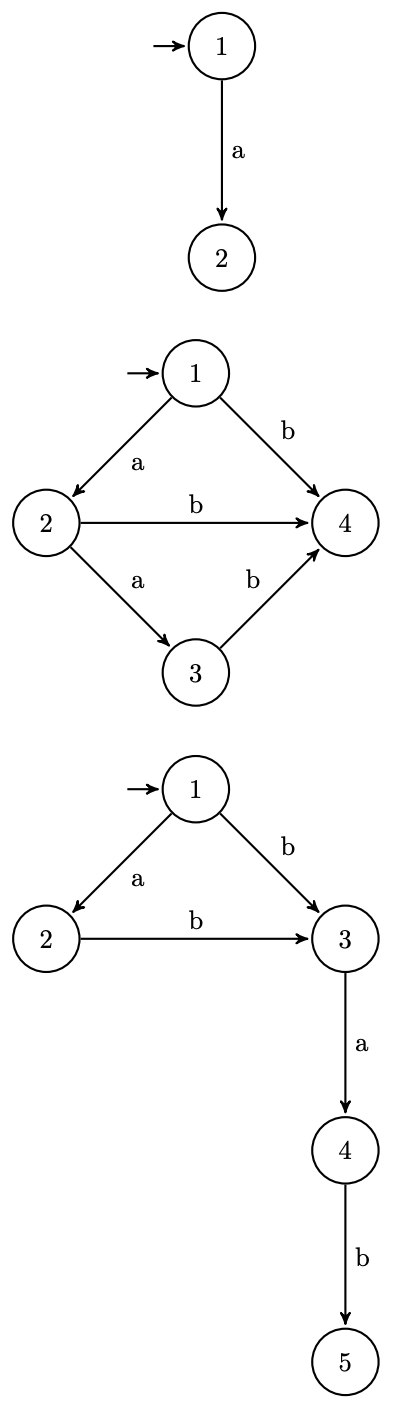시간 제한메모리 제한제출정답맞힌 사람정답 비율
2 초 (추가 시간 없음) 512 MB0000.000%

## 문제

Oleksandr is a fan of strings. His favourite data structure is suffix automaton.

Consider a string $s$ of lowercase English letters. The suffix automaton of $s$ is the smallest (having the smallest number of vertices) directed acyclic graph $G$ with a letter $l(e)$ written on each edge $e$ and a fixed starting vertex $v_0$ such that $$\{w\, |\, w \text{ is a substring of } s\} = \{l(e_1)l(e_2)...l(e_k)\, |\, (e_1, e_2, ..., e_k) \text{ is a path in } G \text{ starting at } v_0\}\text{.}$$

Oleksandr likes suffix automata more than any other graphs. He calls a directed acyclic graph $G$ $s$-beautiful if it is possible to write a lowercase English letter on each edge and choose a starting vertex $v_0$ so that $G$ will become a suffix automaton of the string $s$. Oleksandr likes lexicographically small strings, so please help him find for a given graph $G$ the lexicographically smallest string $s$ such that $G$ is $s$-beautiful.

## 입력

The first line contains two integers $n$ and $m$ ($1 \le n \le 2000$, $1 \le m \le 3000$), the number of vertices and the number of edges in $G$ respectively. Each of the next $m$ lines contains two integers $v$ and $u$ ($1 \le v, u \le n$, $v \ne u$), denoting a directed edge from $v$ to $u$. It is guaranteed that $G$ is acyclic.

## 출력

Output a single line containing the lexicographically smallest string $s$ consisting of lowercase English letters such that $G$ is $s$-beautiful. If such string does not exist, output $-1$.

## 예제 입력 1

2 1
1 2


## 예제 출력 1

a


## 예제 입력 2

4 5
1 2
2 3
1 4
2 4
3 4


## 예제 출력 2

aab


## 예제 입력 3

5 5
1 2
1 3
2 3
3 4
4 5


## 예제 출력 3

abab


## 예제 입력 4

4 5
1 2
1 3
1 4
2 3
4 3


## 예제 출력 4

-1


## 노트

Suffix automata for the first three sample tests are shown below: